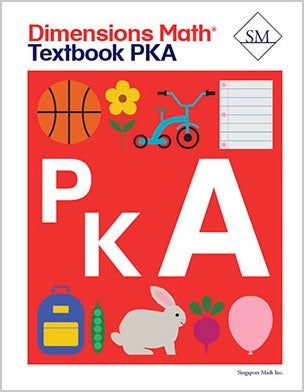# Dimensions Math Textbook PreK-A

\$ 12.00

Chapter 1: Match, Sort, and Classify

Lesson 1: Red and Blue
Lesson 2: Yellow and Green
Lesson 3: Color Review
Lesson 4: Soft and Hard
Lesson 5: Rough, Bumpy, and Smooth
Lesson 6: Sticky and Grainy
Lesson 7: Size — Part 1
Lesson 8: Size — Part 2
Lesson 9: Sort Into Two Groups
Lesson 10: Practice

Chapter 2: Compare Objects

Lesson 1: Big and Small
Lesson 2: Long and Short
Lesson 3: Tall and Short
Lesson 4: Heavy and Light
Lesson 5: Practice

Chapter 3: Patterns

Lesson 1: Movement Patterns
Lesson 2: Sound Patterns
Lesson 3: Create Patterns
Lesson 4: Practice

Chapter 4: Numbers to 5 — Part 1

Lesson 1: Count 1 to 5 — Part 1
Lesson 2: Count 1 to 5 — Part 2
Lesson 3: Count Back
Lesson 4: Count On and Back
Lesson 5: Count 1 Object
Lesson 6: Count 2 Objects
Lesson 7: Count Up to 3 Objects
Lesson 8: Count Up to 4 Objects
Lesson 9: Count Up to 5 Objects
Lesson 10: How Many? — Part 1
Lesson 11: How Many? — Part 2
Lesson 12: How Many Now? — Part 1
Lesson 13: How Many Now? — Part 2
Lesson 14: Practice

Chapter 5: Numbers to 5 — Part 2

Lesson 1: 1, 2, 3
Lesson 2: 1, 2, 3, 4, 5 — Part 1
Lesson 3: 1, 2, 3, 4, 5 — Part 2
Lesson 4: How Many? — Part 1
Lesson 5: How Many? — Part 2
Lesson 6: How Many Do You See?
Lesson 7: How Many Do You See Now?
Lesson 8: Practice

Chapter 6: Numbers to 10 — Part 1

Lesson 1: 0
Lesson 2: Count to 10 — Part 1
Lesson 3: Count to 10 — Part 2
Lesson 4: Count Back
Lesson 5: Order Numbers
Lesson 6: Count Up to 6 Objects
Lesson 7: Count Up to 7 Objects
Lesson 8: Count Up to 8 Objects
Lesson 9: Count Up to 9 Objects
Lesson 10: Count Up to 10 Objects — Part 1
Lesson 11: Count Up to 10 Objects — Part 2
Lesson 12: How Many?
Lesson 13: Practice

Chapter 7: Numbers to 10 — Part 2

Lesson 1: 6
Lesson 2: 7
Lesson 3: 8
Lesson 4: 9
Lesson 5: 10
Lesson 6: 0 to 10
Lesson 7: Count and Match — Part 1
Lesson 8: Count and Match — Part 2
Lesson 9: Practice

Dimensions Math® PreK-5 series features the progression, rigor, and pacing that define Singapore math. Throughout the series, five characters offer students suggestions on how to think about problems. They remind students of strategies they’ve learned and point out important information that encourages them to come up with their own solutions.

Textbook lessons begin with a task that allows students to apply their previous knowledge and learn through discussion. Once students have mastered a concept with the use of concrete and pictorial aids, they are ready to take on more abstract mathematical problem sets. They reach fluency by collecting various strategies along the way and applying them to new problems. Word problems give students a sense of math in real-world contexts.

Workbooks offer independent practice that follows a careful progression of exercise variation. Each textbook lesson includes a corresponding workbook exercise that starts with pictorial representation and progresses to more challenging abstract problems. Workbooks for PreK-2 are perforated.

Teacher’s Guides include lesson plans, mathematical background, games, helpful suggestions, and comprehensive resources for daily lessons. Lessons are laid out clearly and activities are designed for the whole class, small groups, and extension.

Textbooks and Workbooks do not include answer keys. Answers are in Teacher's Guides.
Textbook:
ISBN 9781947226005
pp 144